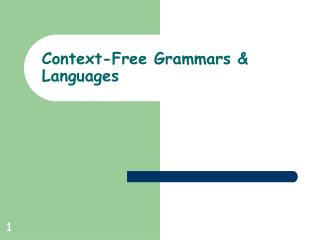# Context-Free Grammars & Languages - PowerPoint PPT PresentationDownload PresentationContext-Free Grammars & Languages

Context-Free Grammars & Languages
Download Presentation## Context-Free Grammars & Languages

- - - - - - - - - - - - - - - - - - - - - - - - - - - E N D - - - - - - - - - - - - - - - - - - - - - - - - - - -
##### Presentation Transcript

1. Context-Free Grammars & Languages

2. Example <program> -> <stmt> <program> | 𝜆 <stmt> -> <assign>|<if>|<while> |… <assign> -> x = 5 | x = 10 <if> -> if (<cond>) <block-stmt> | if (<cond>) <block-stmt> else <block-stmt> | … <cond> -> x == 10 | flag | … <block-stmt> -> <stmt><block-stmt> | 𝜆 …

3. Definition A context-free grammar G = (V, , S, P), where each rule in P has the form A  x • A is a variable (A  V) • x is a string of variables and terminals (x  (V  )* )

4. Language of CFG • Given a CFG G = (V, , S, P), w ∊ L(G) iff w ∊ * and S =>* w • L(G) = { w ∊ * | S =>* w }

5. Context Free Languages A language L is context-free iff There exists a CFG G such that L(G) = L

6. Parsing • Given a grammar G and string w find derivation of w in G (if it exists.)

7. Derivations and Parse Trees • Left-derivation: Always replace the left-most variable • Right-derivation: Always replace the right-most variable • Parse tree: Display derivation in a tree • Root is start variable • Node’s children are symbols of rule used in derivation

8. Ambiguity • A CFG G is ambiguous if • There exists w ∊ L(G) such that • There is more than 1 parse tree for 2 in G. • A language L is inherently ambiguous if • For all CFG G, where L(G) = L, • G is an ambiguous grammar

9. Tasks • Remove ambiguity from grammars where possible • Efficient creation of parse trees